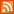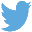Bucaro TecHelpHTTPS Encryption not required because no account numbers orpersonal information is ever requested or accepted by this siteCustom Search# JavaScript Operators

An Operator is a charactor or several charactors that cause JavaScript to perform an operation on a value or variable and returns the result of that operation.

Assignment Operators

"Assignment" is when the code actually places a value into a variable. For example the equal sigh below is used to assign the value 2 to the variable nNum.

```nNum = 2;
```

If you want to confuse non-programmers, you can use special shorthand assignment operators that perform a mathematical operation and assign a value at the same time. For example, the code below assigns the value of nNum + 2 to nNum. The value of nNum below will be 5.

```nNum = 3;
nNum += 2;
```

The code below does exactly the same thing.

```nNum = 3;
nNum = nNum + 2;
```
 Operator Alternate Code nNum -= 3; nNum = nNum - 3; nNum *= 3; nNum = nNum * 3; nNum ⁄= 3; nNum = nNum / 3; nNum %= 3; nNum = nNum % 3;

Comparison Operators

"Comparison" operators compare the values of two variables or statements and return the result of the comparison as either true or false.

 Operator Comparison > greater than < less than == equal to != not equal to <= less than or equal to >= greater than or equal to

The result of the comparison can be used to control the flow of the program. The if ⁄ else structure is the most commonly used control structure.

```nPrice = 29.00;
nHighest = 32.00;

if(nPrice < nHighest)
{
}
else
{
 .menubtn { margin-top:10px; margin-left:50px; width:220px; opacity:1; border-style:solid; border-color:#009000; background-color:#66ff66; transition-property: opacity; transition-duration: 1s; transition-timing-function: ease-out; } .menubtn:hover { opacity:1; background-color:#e5ff23; } Menu - More Java Script Quick ReferenceRSS FeedFollow @Stephen Bucaro